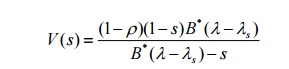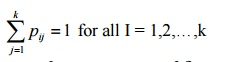Home | | Probability and Queueing Theory | Important Short Objective Questions and Answers: Non-Markovian Queues and Queue Networks

# Important Short Objective Questions and Answers: Non-Markovian Queues and Queue Networks

Mathematics (maths) - Advanced Queueing Models - Important Short Objective Questions and Answers: Non-Markovian Queues and Queue Networks

Non- Markovian Queues and Queue Networks

1. Write down pollaczek-Khintchine formula and explain the notations .

If T is the random service time, the average number of customers in the systemM/G/1 queueing system is markovian comment on this statement.

M/G/1 queueing system is a non markovian queue model. Since the service time follows general distribution.

2.     What do you mean by regeneration points in ( M/G/1 ) model?

The point at which the nth units completes his service and leaves the system.

3.Write down pollaczek-Khintchine transform formula.4. In ( M/G/1 ) model write the formula for the average in the system.Queue Networks

5.     Write classification of Queueing Networks.

¬ Open Networks

¬ Closed Networks

¬ Mixed Network

7.State arrival theorem .

In the closed networks system with m customers, the system as seen by arrivals to server j is distributed as the stationary distribution in the same network system when there are only m-1 customers.

8. Distinguish between open and closed network.

Open Networks

1. Arrivals from outside to the node i(ri ) is allowed.

2. Once the customer gets the service completed at node i, he joins the queue at node j with probability pij or leaves the system with probability pi0

Closed Networks

1.       New customer never enter in to the system.

2.       Existing customer never depart from         the system (ie) pi0 = 0 and ri = 0 or all I (OR) No c ustomer may leave the system.

9. Explain (series queue) tandem queue model.

A series queue or a tandem queue model is satisfies the following characteristics.

¬ Customers may arrive from outside the system at any node and may leave the system from any node.

¬ Customers may enter the system at some node, traverse from node to node in the system and leave the system from some node, necessarily following the same order of nodes.

¬ Customers may return to the nodes aiready visited, skip some nodes and even choose to remain in the system for ever.

10.Define an open Jackson network.

Suppose a queueing network consists of k nodes is called an open Jackson network, if it satisfies the following characteristics.

¬  Customers arriving at node k from outside the system arrive in a Poission pattern with the average arrival rate ri and join the queue at I and wait for his turn for service.

¬ Service times at the channels at node I are independent and each exponentially distributed wite parameter µ .

¬ Once a customer gets the service completed at node i, he joins the queue at node j with probability pij ( whatever be the number of customers waiting at j for service),

when i = 1,2,…,k and j = 1,2,…,k pi0 represents the probability that a customer leaves the system from the i after getting the service at i

¬ The utilization of all the queue is less than one.

11.Define a closed Jackson network.

A queueing network of k nodes is called a closed Jackson network, if new customer never enter into and the existing customer never depart from the system. Viz. if ri = 0 and pi0 for all i.In other words, it is equivalent to a finite source queueing system of N customers who traverse continuously inside the network where the service i is exponentially distributed with rate µi , I =1,2,…,k

The customer completes the service at S j ,j= 1,2,…,k with probability pij where it is assumed that12.What is meant by queue network?

A network of queues is a collection of service centers, which represent system resources, and customers, which represent users or transactions.

13. What do you mean by M/G/1 queue.

In the M/G/1Queueing system under study , we consider a single-server queueing system with infinite capacity, poission arrivals and general service discipli8ne.The model has arbitrary service time, and it is not necessary to be memoryless. Ie .it is not exponentiasl.

14. Write down the formula for the steady-state probability P(n1,n2 ,...,nk ) for multiple server Jackson’s closed network.Study Material, Lecturing Notes, Assignment, Reference, Wiki description explanation, brief detail
Mathematics (maths) : Advanced Queueing Models : Important Short Objective Questions and Answers: Non-Markovian Queues and Queue Networks |